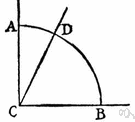# complementary angles

Also found in: Thesaurus, Encyclopedia, Wikipedia.

## complementary angles

pl.n.
Two angles whose sum is 90°.

## com·ple·men·ta·ry angles

(kŏm′plə-mĕn′tə-rē)
Two angles whose sum is 90°.
 Noun 1complementary angles - two angles whose sum is a right angleangle - the space between two lines or planes that intersect; the inclination of one line to another; measured in degrees or radians